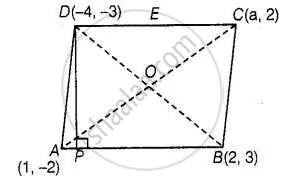# If the points A(1, –2), B(2, 3) C(a, 2) and D(– 4, –3) form a parallelogram, find the value of a and height of the parallelogram taking AB as base. - Mathematics

Sum

If the points A(1, –2), B(2, 3) C(a, 2) and D(– 4, –3) form a parallelogram, find the value of a and height of the parallelogram taking AB as base.

#### Solution

In parallelogram, we know that, diagonals are bisects each other i.e., mid-point of AC = mid-point of BD⇒ ((1 + a)/2, (-2 + 2)/2) = ((2 - 4)/2, (3 - 3)/2)

⇒ (1 + a)/2 = (2 - 4)/2 = (-2)/2 = -1

["Since, midpoint of a line segment having points" (x_1, y_1)  "and"  (x_2, y_2)  "is" ((x_1 + x_2)/2, (y_1 + y_2)/2)]

⇒ 1 + a = -2

⇒ a = -3

So, the required value of a is – 3.

Given that, AS as base of a parallelogram and drawn a perpendicular from D to AS which meet AS at P.

So, DP is a height of a parallelogram.

Now, equation of base AB, passing through the points (1, -2) and (2, 3) is

⇒ (y - y_1) = (y_2 - y_1)/(x_2 - x_1) (x - x_1)

⇒ (y + 2) = (3 + 2)/(2 - 1) (x - 1)

⇒ (y + 2) = 5(x - 1)

⇒ 5x - y = 7  ......(i)

Slope of AB, say m_1 = (y_2 - y_1)/(x_2 - x_1) = (3 + 2)/(2 - 1) = 5

Let the slope of DP be m2.

Since, DP is perpendicular to AB.

By condition of perpendicularity,

m_1 * m_2 = -1 ⇒ 5 * m_2 = -1

⇒ m_2 = -1/5

Now, equation of DP, having slope (-1/5) and passing the point (-4, -3) is

(y - y_1) = m_2(x - x_1)

⇒ (y + 3) = - 1/5(x + 4)

⇒ 5y + 15 = - x - 4

⇒ x + 5y = - 19  .....(ii)

On adding equations (i) and (ii), then we get the intersection point P.

Put the value of y from equation (i) in equation (ii), we get

x + 5(5x - 7) = - 19   .....[Using equation (i)]

⇒ x + 25x - 35 = - 19

⇒ 26x = 16

∴ x = 8/13

Put the value of x in equation (i), we get

y = 5(8/13) - 7 = 40/13 - 7

⇒ y = (40 - 91)/13

⇒ y = (-51)/13

∴ Coordinates of point P = (8/13, (-51)/13)

So, length of the height of a parallelogram,

DP = sqrt((8/13 + 4)^2 + ((-51)/13 + 3)^2

[because "By distance formula, distance between two points"  (x_1, y_1)  "and"  (x_2, y_2)  "is"  d = sqrt((x_2 - x_1)^2 + (y_2 - y_1)^2)]

⇒ DP = sqrt((60/13)^2 + ((-12)/13)^2

= 1/13 sqrt(3600 + 144)

= 1/13 sqrt(3744)

= (12sqrt(26))/13

Hence, the required length of height of a parallelogram is (12sqrt(26))/13.

Concept: Distance Formula
Is there an error in this question or solution?

#### APPEARS IN

NCERT Mathematics Exemplar Class 10
Chapter 7 Coordinate Geometry
Exercise 7.4 | Q 4 | Page 86